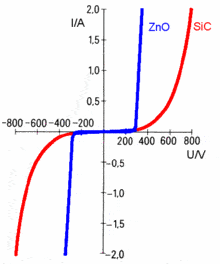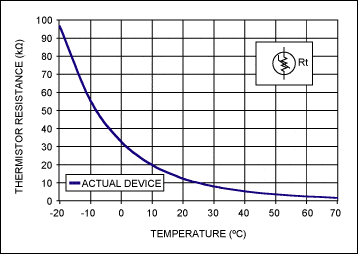# Non-Ohmic Materials

So I learned today about the version of Ohm's law where

$$J = \sigma E$$

and my professor mentioned that some materials (non-Ohmic materials like superconductors) have "vanishingly small" extra terms that are quadratic or cubic. I'm wondering if anyone can direct me to a source (or even just tell me) where I can find out just how small these terms are for Ohmic (linearly conducting) materials.

berkeman
Mentor
So I learned today about the version of Ohm's law where

$$J = \sigma E$$

and my professor mentioned that some materials (non-Ohmic materials like superconductors) have "vanishingly small" extra terms that are quadratic or cubic. I'm wondering if anyone can direct me to a source (or even just tell me) where I can find out just how small these terms are for Ohmic (linearly conducting) materials.

The traditional example of non-ohmic materials are semiconductors and semiconductor junctions. The V-I curve of a p-n junction diode is exponential in nature.

But it sounds like you are asking for examples of slight non-linearities in simple conductors? I suppose if the current density is high enough, the transient heating effects might be considered a non-linearity. But the material is still Ohmic, if you use the resistivity for the temperature at each point in the conductor...

Svein
For example, the Voltage Dependent Resistor that we use for damping voltage spikes.Or the Negative Temperature Coefficient Resistor (NTC)- the resistance decreases when the temperature increases. Since the current through the NTC will generate heat, it can be used to limit "inrush current".You also have the Positive Temperature Coefficient Resistor (PTC)- the resistance increases when the temperature increases. Since the current through the PTC will generate heat, it can be used to generate a startup-current that gradually decreases (for example if you want to demagnetize an instrument when you turn it on).
http://www9.dw-world.de/rtc/infotheque/electronic_components/fig1521.gif [Broken]

Last edited by a moderator:
Thank you both.